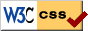# Notes on Diffy Qs - SAGE demonstrations for section 1.7

Press the Activate button below to launch the SAGE demonstration. After the sage cell is evaluated you should be able to interact with it to change numbers with sliders. You may have to wait a little before the graph appears. Be patient. Below the demo is an area for experimenting with SAGE and Euler's method.

## Euler's method demo

Euler's method for

$y' = 5x + y - 5$, $y(0) = 1$,

using step size h. The actual solution is also plotted to compare. The slider will modify the step size.

## Euler's method with SAGE

First let's just do the table

Now let's plot the result.

How about plotting the actual solution. Here SAGE will only plot the solution if it can find the actual solution analytically.

The code is mainly due to Ryan Burkhart, it was then slightly modified by Jiří Lebl.